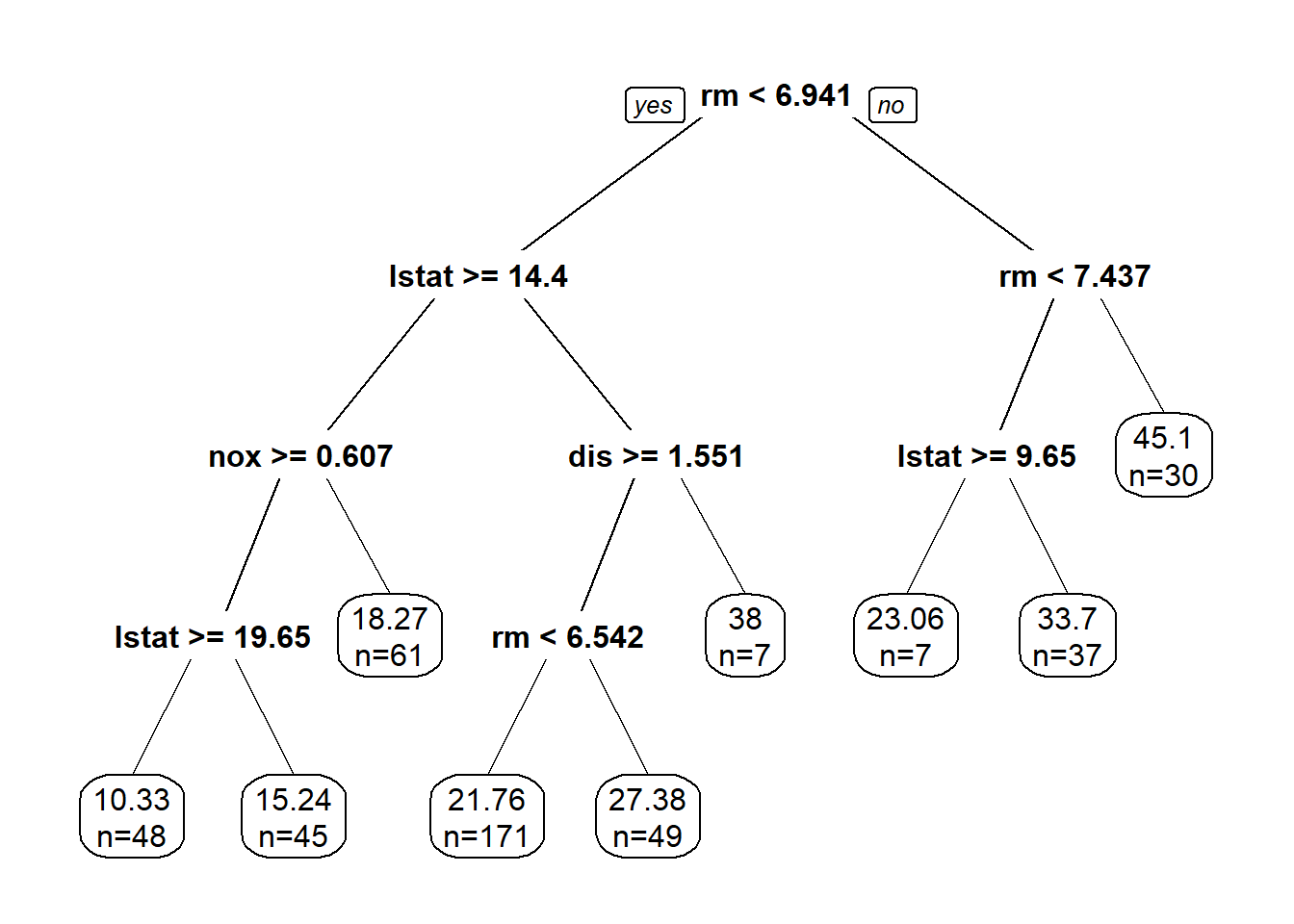# 1Exercise: How to interpret the tree model!

library(MASS) #this data is in MASS package
sample_index <- sample(nrow(Boston),nrow(Boston)*0.90)
boston_train <- Boston[sample_index,]
boston_test <- Boston[-sample_index,]
library(rpart)
library(rpart.plot)
boston_rpart <- rpart(formula = medv ~ ., data = boston_train)
prp(boston_rpart,digits = 4, extra = 1)1. Based on the tree model above, what is the predicted median housing price (in thousand) given following information:
crim zn indus chas nox rm age dis rad tax ptratio black lstat medv
0.13 25 5.13 0 0.45 6.76 43.4 7.98 8 284 19.7 395.58 9.5 25
1. Calculate the mean squared error (MSE) of the in-sample for this tree model;
1. Compare this model’s out-of-sample performance with the linear regression model with all variables in it.

go to top